Next: Testing for nonlinearity Up: Information dimension Previous: Information dimension

### Entropy estimates

The correlation dimension characterizes thedependence of the correlation sum inside the scaling range. It is natural to ask what we can learn form its m-dependence, once m is larger than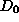. The number of-neighbors of a delay vector is an estimate of the local probability density, and in fact it is a kind of joint probability: All m-components of the neighbor have to be similar to those of the actual vector simultaneously. Thus when increasing m, joint probabilities covering larger time spans get involved. The scaling of these joint probabilities is related to the correlation entropy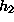, such that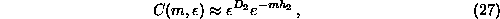As for the scaling in, also the dependence on m is valid only asymptotically for large m, which one will not reach due to the lack of data points. So one will study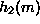versus m and try to extrapolate to large m. The correlation entropy is a lower bound of the Kolmogorov Sinai entropy, which in turn can be estimated by the sum of the positive Lyapunov exponents. The program d2 produces as output the estimates ofdirectly, from the other correlation sum programs it has to be extracted by post-processing the output.

The entropies of first and second order can be derived from the output of c1 and d2 respectively. An alternate means of obtaining these and the other generalized entropies is by a box counting approach. Let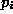be the probability to find the system state in box i, then the order q entropy is defined by the limit of small box size and large m of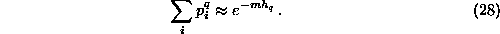To evaluate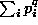over a fine mesh of boxes in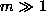dimensions, economical use of memory is necessary: A simple histogram would take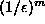storage. Therefore the program boxcount implements the mesh of boxes as a tree with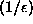-fold branching points. The tree is worked through recursively so that at each instance at most one complete branch exists in storage. The current version does not implement finite sample corrections to Eq.().Next: Testing for nonlinearity Up: Information dimension Previous: Information dimension

Thomas Schreiber
Wed Jan 6 15:38:27 CET 1999